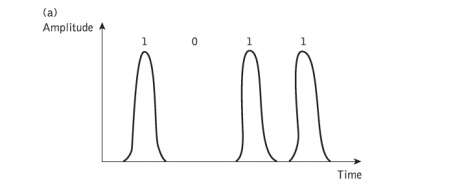# Dispersion

Dispersion of the transmitted optical signal causes distortion for both digital and analog transmission along optical fibers.

Dispersion

Dispersion of the transmitted optical signal causes distortion for both digital and analog transmission along optical fibers. When considering the major implementation of optical fiber transmission which involves some form of digital modulation, then dispersion mechanisms within the fiber cause broadening of the transmitted light pulses as they travel along the channel. The phenomenon is illustrated in Figure 2.7, where it may be observed that each pulse broadens and overlaps with its neighbors, eventually becoming indistinguishable at the receiver input. The effect is known as intersymbol interference (ISI). Thus an increasing number of errors may be encountered on the digital optical channel as the ISI becomes more pronounced. The error rate is also a function of the signal attenuation on the link and the subsequent signal-to-noise ratio (SNR) at the receiver.

For no overlapping of light pulses down on an optical fiber link the digital bit rate BT must be less than the reciprocal of the broadened (through dispersion) pulse duration (2t).

Hence:The conversion of bit rate to bandwidth in hertz depends on the digital coding format used. For metallic conductors when a nonreturn-to-zero code is employed, the binary 1 level is held for the whole bit period τ. In this case there are two bit periods in one wavelength (i.e. 2 bits per second per hertz), as illustrated in Figure 2.8(a). Hence the maximum bandwidth B is one-half the maximum data rate or:However, when a return-to-zero code is considered, as shown in Figure 2.8(b), the binary 1 level is held for only part (usually half ) of the bit period. For this signaling scheme the data rate is equal to the bandwidth in hertz (i.e. 1 bit per second per hertz) and thus BT = B.The bandwidth B for metallic conductors is also usually defined by the electrical 3 Db points (i.e. the frequencies at which the electric power has dropped to one-half of its constant maximum value). However, when the 3 dB optical bandwidth of a fiber is considered it is significantly larger than the corresponding 3 dB electrical bandwidth. Hence, when the limitations in the bandwidth of a fiber due to dispersion are stated (i.e. optical bandwidth Bopt), it is usually with regard to a return to zero code where the bandwidth in hertz is considered equal to the digital bit rate. Within the context of dispersion the bandwidths expressed in this chapter will follow this general criterion unless otherwise stated.

when electro-optic devices and optical fiber systems are considered it is more usual to statethe electrical 3 dB bandwidth, this being the more useful measurement when interfacing an optical fiber link to electrical terminal equipment.

Study Material, Lecturing Notes, Assignment, Reference, Wiki description explanation, brief detail
Optical Communication and Networking : Transmission Characteristics of Optical Fiber : Dispersion |